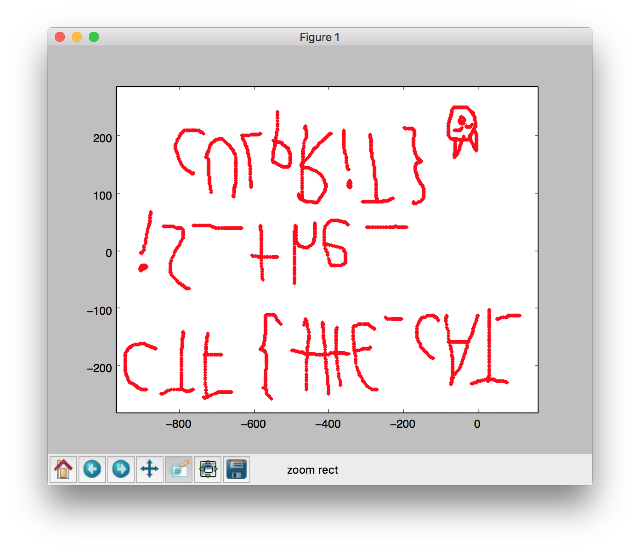# Google Capture The Flag 2016) Forensic-For2 Write up

The Google Capture The Flag 2016 was run on the 2016.04.29 ~ 30 (48h).
This is write up about the forensics “For2” which was 200 points.

In For2, capture.pcapng was provided but there was any description.
Anyway i was given a pcap file.

After opening the file in Wireshark, it looked like a USB capture.

The majority of the "URB Function" shows
“TERRUPT_TRANSFERURB_FUNCTION_BULK_OR_INTERRUPT_TRANSFER”
And these packet have some data.

When i check on the first instance of source 1.3.0
It appears to be a Logitech Optical Mouse, as shown below.
I tried to export raw data for mouse event from pcapng file.
``````h2spices-MacBook-Pro:tmp h2spice\$ tshark -r capture.pcapng -Y 'usb.data_len == 4' -T fields -e usb.capdata > mouse_event
h2spices-MacBook-Pro:tmp h2spice\$ tail ./mouse_event
00:fb:00:00
00:fc:00:00
00:fc:ff:00
00:fe:00:00
00:fe:ff:00
00:fe:00:00
00:ff:00:00
00:fe:ff:00
00:ff:ff:00
01:00:00:00
``````

Raw data can be converted to coordinates.
(here is useful code https://johnroach.info/2011/02/16/getting-raw-data-from-a-usb-mouse-in-linux-using-python/)
``````#!/usr/bin/python

filename = "mouse_event"

def to_signed(h):
i = int(h, 16)
return i - ((0x80 & i) << 1)

coordinate_x = 0
coordinate_y = 0

if len(line) > 1:
status, raw_x, raw_y, junk = line.split(":")
coordinate_x += to_signed(raw_x)
coordinate_y += to_signed(raw_y)

if status != "00":
print "%d %d" % (coordinate_x, coordinate_y)

``````
output is as shown below.
``````h2spices-MacBook-Pro:tmp h2spice\$ python convert_raw2coordinates.py
-273 -428
-889 -242
-890 -241
-891 -241
-892 -241
-893 -241
-894 -241
-897 -241
-898 -241
-899 -241
-901 -241
-902 -241
-904 -240
-906 -240
-907 -240
-909 -239
-910 -238
-911 -238
-912 -238
-913 -237
-914 -236
-915 -235
-916 -235
-917 -235
[...]
``````

I drew graph using 'pyplot' because there were so many coordinates.
``````#!/usr/bin/python

import sys
import matplotlib.pyplot as plt
plt.xlim(-1000, 1000)
plt.ylim(-1000, 1000)

filename = "mouse_event"

def to_signed(h):
i = int(h, 16)
return i - ((0x80 & i) << 1)

coordinate_x = 0
coordinate_y = 0

if len(line) > 1:
status, raw_x, raw_y, junk = line.split(":")
coordinate_x += to_signed(raw_x)
coordinate_y += to_signed(raw_y)

if status != "00":
print "%d %d" % (coordinate_x, coordinate_y)
plt.plot(coordinate_x, coordinate_y, color="red", marker=".")

plt.show()
``````Flag is CTF{tHE_cAT_iS_the_cULpRiT}

If you need my help, tell me anytime. Facebook